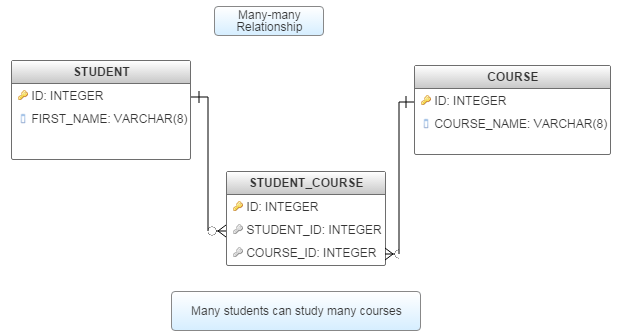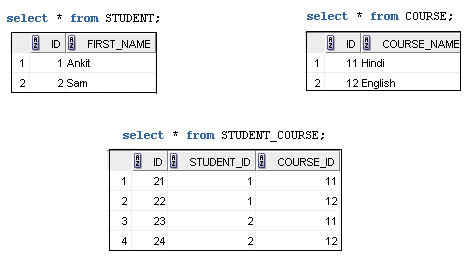### Many-Many relationship in MongoDB

You are here : Home / MongoDB Tutorial

Contents of page >
• This is the most normalized and best form to represent many to many relationship data model.
• STEP 1.1) create and insert in STUDENT collection >
• STEP 1.2) create and insert in COURSE collection >
• STEP 1.3) create and insert in STUDENT_COURSE collection >
• 2.1) Many-Many Relationship - Table structure in RDBMS >
• 2.2) Sql script to create above tables in RDBMS (in oracle) >
• 2.2.3) Let’s see tables after inserting data in RDBMS >
• 3 > Now, let’s read/query/find in above (2.1) MongoDB collections >

A) (Many-to-Many) Many-to-Many Relationships with Document Reference
This is the most normalized and best form to represent many to many relationship data model.
Create student collection and COURSE collection.
Then create STUDENT_COURSE collection which contains reference to student and COURSE collection.
1) Create collections in MongoDB >

STEP 1.1) create and insert in STUDENT collection >
 db.STUDENT.insert({  "_id": 1,  "FIRST_NAME": "Ankit"}) db.STUDENT.insert({  "_id": 2,  "FIRST_NAME": "Sam"})

STEP 1.2) create and insert in COURSE collection >
 db.COURSE.insert({  "_id": 11,  "COURSE_NAME": "Hindi"}) db.COURSE.insert({  "_id": 12,  "COURSE_NAME": "English"})

STEP 1.3) create and insert in STUDENT_COURSE collection >
 db.STUDENT_COURSE.insert({  "_id": 21,  "STUDENT_ID": 1 ,  "COURSE_ID": 11}) db.STUDENT_COURSE.insert({  "_id": 22,  "STUDENT_ID": 1 ,  "COURSE_ID": 12}) db.STUDENT_COURSE.insert({  "_id": 23,  "STUDENT_ID": 2 ,  "COURSE_ID": 11}) db.STUDENT_COURSE.insert({  "_id": 24,  "STUDENT_ID": 2 ,  "COURSE_ID": 12,})

2) Now, let’s see above many-many relationship of mongoDB collections in RDBMS (relational database) >

2.1) Many-Many Relationship - Table structure in RDBMS >2.2) Sql script to create above tables in RDBMS (in oracle) >
 create table STUDENT (ID number PRIMARY KEY,                    FIRST_NAME varchar2(22)); create table COURSE (ID number PRIMARY KEY,                    COURSE_NAME varchar2(22) ); create table STUDENT_COURSE (ID number PRIMARY KEY,                    STUDENT_ID number,                    COURSE_ID number,                    FOREIGN KEY (STUDENT_ID) REFERENCES STUDENT (ID),                    FOREIGN KEY (COURSE_ID) REFERENCES COURSE (ID) );

2.2.3) Let’s see tables after inserting data in RDBMS >Here, Many students study many courses.

3 > Now, let’s read/query/find in above (2.1) MongoDB collections >

Query 3.1 > Query to find and show all students
 db.STUDENT.find().pretty()
Output>
 { "_id" : 1, "FIRST_NAME" : "Ankit" } { "_id" : 2, "FIRST_NAME" : "Sam" }

Query 3.2 > Query to find and show all students
 db.COURSE.find().pretty()
Output>
 { "_id" : 11, "COURSE_NAME" : "Hindi" } { "_id" : 12, "COURSE_NAME" : "English" }

Query 3.3 > Query to find and show all student_courses
 db.STUDENT_COURSE.find().pretty()
Output>
 { "_id" : 21, "STUDENT_ID" : 1, "COURSE_ID" : 11 } { "_id" : 22, "STUDENT_ID" : 1, "COURSE_ID" : 12 } { "_id" : 23, "STUDENT_ID" : 2, "COURSE_ID" : 11 } { "_id" : 24, "STUDENT_ID" : 2, "COURSE_ID" : 12 }

B) Now let’s cover above point - (Inserting related documents in same collection) - Many-to-Many Relationships with Document Reference >

create and insert in STUDENT collection (Also insert related data i.e. data (documents) of COURSE and STUDENT_COURSE in STUDENT collection) >
 db.STUDENT.insert({  "_id": 1,  "FIRST_NAME": "Ankit"}) db.STUDENT.insert({  "_id": 2,  "FIRST_NAME": "Sam"}) db.STUDENT.insert({  "_id": 11,  "COURSE_NAME": "Hindi"}) db.STUDENT.insert({  "_id": 12,  "COURSE_NAME": "English"}) db.STUDENT.insert({  "_id": 21,  "STUDENT_ID": 1 ,  "COURSE_ID": 11}) db.STUDENT.insert({  "_id": 22,  "STUDENT_ID": 1 ,  "COURSE_ID": 12}) db.STUDENT.insert({  "_id": 23,  "STUDENT_ID": 2 ,  "COURSE_ID": 11}) db.STUDENT.insert({  "_id": 24,  "STUDENT_ID": 2 ,  "COURSE_ID": 12,})

C) Summary -

So in this mongoDB tutorial we learned how to create and manage Many-Many relationship in MongoDB.

Having any doubt? or you liked the tutorial! Please comment in below section.